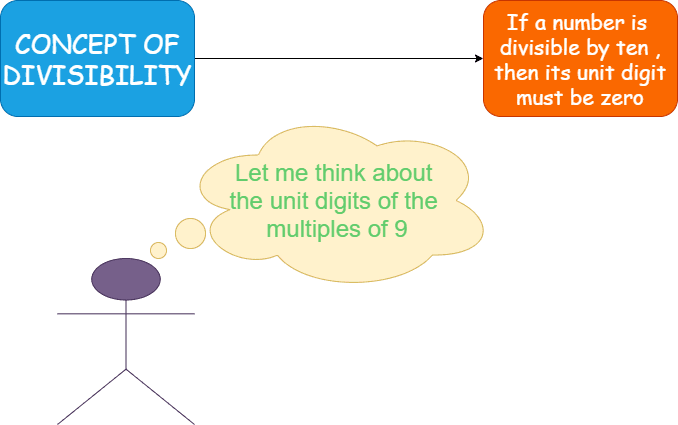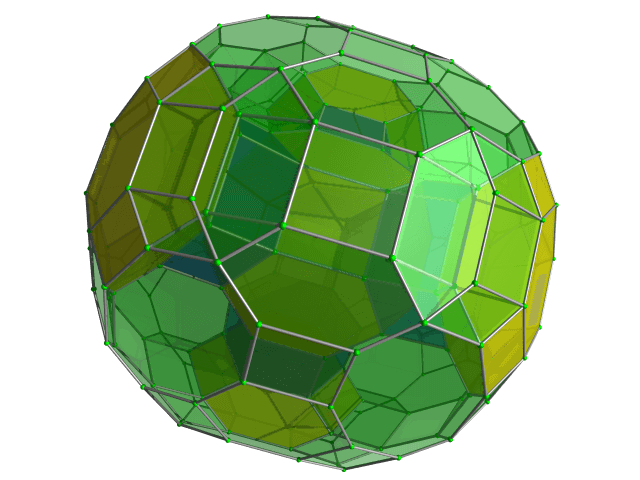Categories

# Divisibility AMC 8, 2016 Problem 5

Try this beautiful problem from AMC 8. It involves the concept of divisibility. We provide sequential hints so that you can try the problem.

## Competency in Focus: Divisibility.

This problem from American Mathematics contest (AMC 8, 2016) is based on the concept of divisibility .

## First look at the knowledge graph.## Next understand the problem

The number $N$ is a two-digit number. • When $N$ is divided by $9$, the remainder is $1$. • When $N$ is divided by $10$, the remainder is $3$. What is the remainder when $N$ is divided by $11$?   $\textbf{(A) }0\qquad\textbf{(B) }2\qquad\textbf{(C) }4\qquad\textbf{(D) }5\qquad \textbf{(E) }7$
##### Source of the problem

American Mathematical Contest 2016, AMC 8  Problem 5

### Divisibility

4/10
##### Suggested Book

Do you really need a hint? Try it first!
When $N$ is divided by $10$ it leaves remainder $3$ i.e., $N=10\times P+3 \textbf{ ,where } P$ is an integer. i.e., The unit digit of $N$ must be $3$ because unit digit of $10P$ is zero.
$N$ leaves remainder $1$ when divided by $9$. i.e., $N=9\times Q+1$ where $Q$ is an integer. Since $10\times P+3=9\times Q+1$ the unit digit of $9\times Q +1$ must be $3$.
Since $N$ is a two digit number then the only possibility is $Q=8$ i.e., $N=9\times 8+1=73$

## AMC - AIME Program

AMC - AIME - USAMO Boot Camp for brilliant students. Use our exclusive one-on-one plus group class system to prepare for Math Olympiad## Pigeonhole Principle

“The Pigeonhole principle” ~ Students who have never heard may think that it is a joke. Pigeonhole Principle is one of the simplest but most useful ideas in mathematics. Let’s learn the Pigeonhole Principle with some applications. Pigeonhole Principle Definition: In...

## External Tangent | AMC 10A, 2018 | Problem 15

Try this beautiful Problem on geometry based on circle from AMC 10A, 2018. Problem-15. You may use sequential hints to solve the problem.

## Dice Problem | AMC 10A, 2014| Problem No 17

Try this beautiful Problem on Probability from AMC 10A, 2014. Problem-17, You may use sequential hints to solve the problem.

## Problem on Curve | AMC 10A, 2018 | Problem 21

Try this beautiful Problem on Co-ordinate geometry from AMC 10A, 2018. Problem-21, You may use sequential hints to solve the problem.

## Right-angled Triangle | AMC 10A, 2018 | Problem No 16

Try this beautiful Problem on triangle from AMC 10A, 2018. Problem-16. You may use sequential hints to solve the problem.

## Finding Greatest Integer | AMC 10A, 2018 | Problem No 14

Try this beautiful Problem on Algebra from AMC 10A, 2018. Problem-14, You may use sequential hints to solve the problem.

## Length of the crease | AMC 10A, 2018 | Problem No 13

Try this beautiful Problem on triangle from AMC 10A, 2018. Problem-13. You may use sequential hints to solve the problem.

## Right-angled shaped field | AMC 10A, 2018 | Problem No 23

Try this beautiful Problem on triangle from AMC 10A, 2018. Problem-23. You may use sequential hints to solve the problem.

## Area of region | AMC 10B, 2016| Problem No 21

Try this beautiful Problem on Geometry on Circle from AMC 10B, 2016. Problem-20. You may use sequential hints to solve the problem.

## Coin Toss Problem | AMC 10A, 2017| Problem No 18

Try this beautiful Problem on Probability from AMC 10A, 2017. Problem-18, You may use sequential hints to solve the problem.

This site uses Akismet to reduce spam. Learn how your comment data is processed.